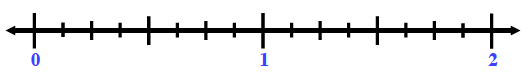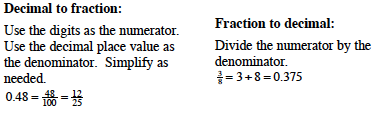### Home > CC1 > Chapter Ch6 > Lesson 6.2.2 > Problem6-88

6-88.

Draw a number line from 0 to 2. Then write the following numbers in its correct place on the number line. Homework Help ✎

 $0.2$ $\frac { 13 } { 26 }$ $1.5$ $\frac { 1 } { 8 }$ $1.9$ $\frac { 7 } { 8 }$ $1.09$ $\frac { 3 } { 5 }$ $1.19$